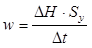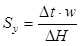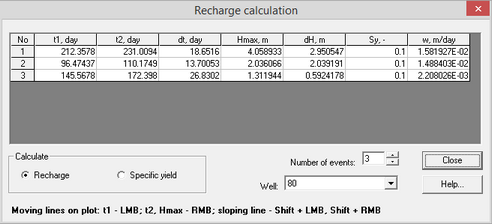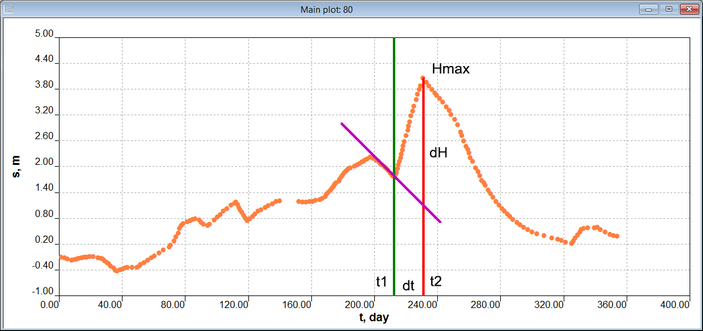﻿ ANSDIMAT+ > Aquifer tests analysis > Determination of recharge

# Recharge

Navigation:  ANSDIMAT+ > Aquifer tests analysis >

# Recharge

The program determines recharge based on its impact on water levels in monitoring boreholes. Recharge rate can be determined for selected individual rainfall events using the method explained in Healy and Cook (2002). Specific yield (Sy) needs to be specified for recharge determination. Alternatively, Sy can be determined, when recharge rate is specified.

The dialog window "Recharge" is opened through menu "Analysis > Recharge". Menu "Recharge" is enabled after selection of conceptual model "Natural fluctuations" and plot "s-t" for observation well(s).

When the dialog window "Recharge" is open, the three lines appear on the plot: two vertical lines and one sloping line. ANSDIMAT calculates recharge based on coordinates and directions of these lines. The lines can be moved by the mouse and key buttons:

green line – clicking left mouse button within the plot window will move the line to the cursor location. This line corresponds to the minimum water level;

red line – clicking red mouse button within the plot window will move the upper end of the red vertical line to the cursor location. The red line is the vertical line from the minimum Y-axis value to the maximum water level (Hmax) that is selected for this analysis;

violet line - determines hydraulic head trend prior to the recharge event. This line can be moved by pressing left and right mouse buttons and SHIFT at the same time. The line must approximate factual measurements prior to the rainfall event.

Recharge rate (w), when specific yield (Sy) is specified,  is calculated by the formula:Specific yield (Sy), when recharge (w) is specified, is calculated by the formula:where Δt – the time interval between the green and the red lines (see figure below); ΔH – Hydraulic head change between Hmax and the point of crossing the red and the violet lines.Dialog window "Recharge calculation"

Explanatory table

 Table Number of rows in the table corresponds to the analyzed number of events for the selected well. Depending on selected option (see Frame "Calculate") recharge rate and specific yield are either calculated using the plot (see the figure below) or entered manually. •Column "No" – sequence number of event;•Column "t1, day" – time of the minimum hydraulic head (i.e. the start of water levels reaction on the selected recharge event). This parameter is either assigned manually or from the plot by the location of the green line. To change location of the green line, left mouse button can be used; •Column "t2, day" – time of the maximum hydraulic head. This parameter is either assigned manually or from the plot by the location of the red line. To change location of the red line, right mouse button can be used; •Column "dt, day" – time interval between the minimum and the maximum hydraulic heads. The value is calculated automatically: dt = t1 – t2;  •Column "Hmax, m" – maximum hydraulic head at the time t2, caused by the recharge event. This parameter is either assigned manually or from the plot by taking coordinates of the upper and of the red line. To change length of the right line, red mouse button can be used;•Column "dH, m" – calculated change of hydraulic head from its specified maximum value Hmax to the point of crossing of the red and the violet lines;•Column "Sy, m" – Specific yield value. The value is entered manually with calculation option "Recharge" and it is calculated, when option "Specific yield" is checked;•Column "w, m/day" – recharge rate. The value is entered manually with calculation option "Specific yield" and it is calculated, when option "Recharge" is checked.  When cursor moves between the table rows, the lines location on the plot change accordingly to a highlighted recharge event. Frame "Calculate" Selects parameter to calculate. Option "Recharge" – calculates recharge rate, requires Specific yield value. Option "Specific yield" – calculates specific yield, requires recharge rate. Field "Number of events" Number of analyzed peak events (between 1 and 999) for the selected observation well. List "Well" Allows selection of an observation well for recharge determination. The selected wells must have plots in s – t coordinates. The results are saved for all selected wells.Example of recharge determination on st plot

References

Healy R.W., Cook P.G. Using groundwater levels to estimate recharge // Hydrogeology Journal. 2002. Vol. 10. P. 91–109".